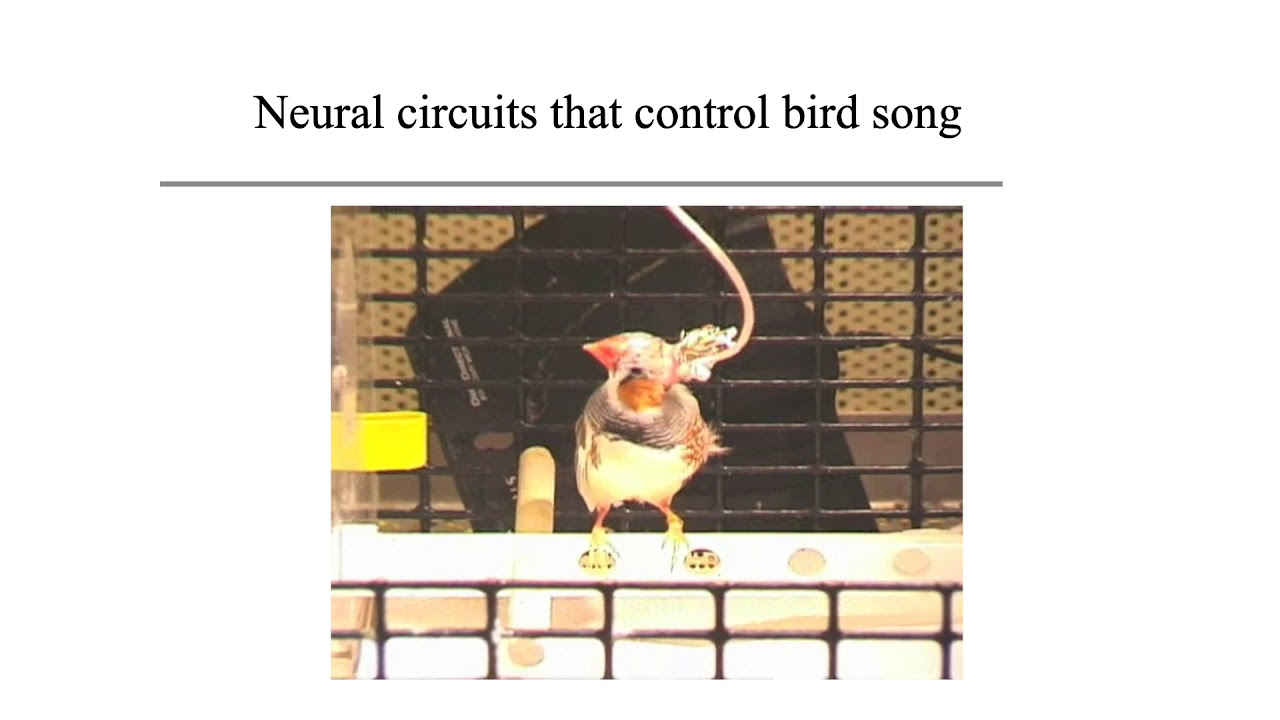MIT OpenCourseWare

# Introduction to Neural Computation

This course introduces quantitative approaches to understanding brain and cognitive functions. Topics include mathematical description of neurons, the response of neurons to sensory stimuli, simple neuronal networks, statistical inference and decision making. It also covers foundational quantitative tools of data analysis in neuroscience: correlation, convolution, spectral analysis, principal components analysis, and mathematical concepts including simple differential equations and linear algebra. (from ocw.mit.edu )

Instructor

Psychology
2020

#### Lecture 01 - Overview and Ionic Currents